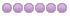× General Purpose Components and Libraries, discussions, problems and suggestions

# Idea Text in TPLRectAngle is written over/beyond right border

• Topic Author
• Offline
• Fresh Boarder
•2 years 10 months ago - 2 years 10 months ago #11011 by Adular
Hello,

i experienced problems with text inside TPLRectangle.
1. random text was additionally written
Solution: initializing rs in procedure TrimLine
2. text was written over/beyond right border in TPLRectAngle
Solution:
procedure TrimLine was modfied
procedure TplRectangle.DrawStringsInRect was modified

Here are my changes, but be warned:
it works for me, but due to the lack of skills, id did no do any intensive testing (sideeffects etc.):

greetings

in file TPLShapeObjects

changed: starting from line 834
Original
```//TrimLine: Splits off from LS any characters beyond the allowed width
//breaking at the end of a word if possible. Leftover chars -> RS.
procedure TrimLine(canvas: TCanvas; var ls: string; out rs: string; LineWidthInPxls: integer);
var
i, len, NumCharWhichFit: integer;
dummy: TSize;
begin
if ls = '' then
exit;
len := length(ls);

{ TODO -oTC -cLazarus_Port_Step2 : find a linux replacement for GeTplTextExtentExPoint }
//TCQ
////get the number of characters which will fit within LineWidth...
//if not GeTplTextExtentExPoint(canvas.handle,
//  pchar(ls),len,LineWidthInPxls,NumCharWhichFit,0,dummy) then
//  begin
//    ls := '';
//    rs := '';
//    exit; //oops!!
//  end;
//TCQ replaced by
NumCharWhichFit := len;

if NumCharWhichFit = len then
exit //if everything fits then stop here
else if NumCharWhichFit = 0 then
begin
rs := ls;
ls := '';
end
else
begin
i := NumCharWhichFit;
//find the end of the last whole word which will fit...
while (NumCharWhichFit > 0) and (ls[NumCharWhichFit] > ' ') do
Dec(NumCharWhichFit);
if (NumCharWhichFit = 0) then
NumCharWhichFit := i;

i := NumCharWhichFit + 1;
//ignore trailing blanks in LS...
while (ls[NumCharWhichFit] = ' ') do
Dec(NumCharWhichFit);
//ignore beginning blanks in RS...
while (i < len) and (ls[i] = ' ') do
Inc(i);
rs := copy(ls, i, len);
ls := copy(ls, 1, NumCharWhichFit);        //nb: assign ls AFTER rs here
end;
end;
```

my changes:
```//TrimLine: Splits off from LS any characters beyond the allowed width
//breaking at the end of a word if possible. Leftover chars -> RS.
procedure TrimLine(canvas: TCanvas; var ls: string; out rs: string; LineWidthInPxls: integer);
const
word_delimiter:string=';,:.+-<>*!"§\$%&/()=?';
var
i, j,len, NumCharWhichFit: integer;
dummy: TSize;
charlen_w: integer; // Char length of widest Character ='W';
charlen_s:integer; // Char length of smallest Character = ''';
maxcount_w:integer; // maximum number of widest character 'W' in linewidthinpixels
maxcount_s:integer; // maximum number of smallest character in linewidthinPixels
pixellen_s, pixellen_w, pixellen_actual,w:integer; // Pixellength of smallest and widest charaters and actual character length

begin
rs:='';  // new
if ls = '' then
exit;
len := length(ls);

{ TODO -oTC -cLazarus_Port_Step2 : find a linux replacement for GeTplTextExtentExPoint }
//TCQ
////get the number of characters which will fit within LineWidth...
//if not GeTplTextExtentExPoint(canvas.handle,
//  pchar(ls),len,LineWidthInPxls,NumCharWhichFit,0,dummy) then
//  begin
//    ls := '';
//    rs := '';
//    exit; //oops!!
//  end;
//TCQ replaced by

charlen_w:=canvas.TextWidth('W');
if charlen_w=0 then charlen_w:=1;    // no division by zero
maxcount_w:=LineWidthInPxls div charlen_w;
if len < maxcount_w then exit;

//NumCharWhichFit := len;
NumCharWhichFit:=maxcount_w;

if NumCharWhichFit > len then        // was  NumCharWhichFit = len
exit //if everything fits then stop here
else if NumCharWhichFit = 0 then
begin
rs := ls;
ls := '';
end
else
begin
charlen_s:=canvas.TextWidth('''');      // width of smallest character
maxcount_s:=LineWidthInPxls div charlen_s;
if maxcount_s=0 then maxcount_s:=1; // no division by zero;

// situation:  len > maxcount_w, so that ls possibly doesn't fit
// maxcount_s is the maximum number of the smallest character, so left(ls,1,maxcount_s) fits
// solution
//  find number of chars which are >= maxcount_w and <= maxcount_s which fits
// left(ls, maxcount_w) should always fit
// left(ls, maxcount_s) should not fit
// starting point: textwidth(left(ls, maxcount_s)) and counting down to maxcount_w
i:=maxcount_s;

pixellen_actual:=canvas.textwidth(copy(ls,1,maxcount_s-1));
while (pixellen_actual > LineWidthInPxls) do begin
w:=canvas.textwidth(copy(ls,i,1));
dec(i);
pixellen_actual:=pixellen_actual-w;
end;
NumCharWhichFit:=i;
j:=i;

// so  left(ls,i) should fit
// looking for delimiters
while (j>0) and (
(ls[j]>chr(32)) and (pos(ls[j],word_delimiter)=0) ) do dec(j);

if j>1 then NumCharWhichFit:=j-1;

{
i := NumCharWhichFit;
//find the end of the last whole word which will fit...
while (NumCharWhichFit > 0) and (ls[NumCharWhichFit] > ' ') do
Dec(NumCharWhichFit);
if (NumCharWhichFit = 0) then
NumCharWhichFit := i;

i := NumCharWhichFit + 1;
//ignore trailing blanks in LS...
while (ls[NumCharWhichFit] = ' ') do
Dec(NumCharWhichFit);
//ignore beginning blanks in RS...
while (i < len) and (ls[i] = ' ') do
Inc(i);
}

//rs := copy(ls, i, len);

rs := copy(ls, NumCharWhichFit+1, len);
ls := copy(ls, 1, NumCharWhichFit);        //nb: assign ls AFTER rs here
end;
end; ```

and starting from line 3099
procedure TplRectangle.DrawStringsInRect(aCanvas: TCanvas; aStrings: TStrings);
```begin
with aCanvas do
begin
lineHeight := TextHeight('Yy');
if odd(Pen.Width) then
XCenter := (BtnPoints.X + BtnPoints.X) div 2;
YLimit := BtnPoints.Y - lineHeight - pad;
space := BtnPoints.X - BtnPoints.X - pad * 2;
CalcOnlyOrTextOut(True);
i := BtnPoints.Y - pad - YPos;
if i > 1 then
Inc(YPos, i div 2);
CalcOnlyOrTextOut(False);
end;
end;
```

changed to:
```begin
ls:='';  // no strange Text anymore
rs:='';
with aCanvas do
begin
lineHeight := TextHeight('Yy');
pad := padding + pen.width+abs(ShadowSize); //(Pen.Width div 2);  changed because text was written right of the border
if odd(Pen.Width) then
XCenter := (BtnPoints.X + BtnPoints.X) div 2;
YLimit := BtnPoints.Y - lineHeight - pad;
space := BtnPoints.X - BtnPoints.X - pad * 2;
CalcOnlyOrTextOut(True);
i := BtnPoints.Y - pad - YPos;
if i > 1 then
Inc(YPos, i div 2);
CalcOnlyOrTextOut(False);
end;
end;     ```
Last edit: 2 years 10 months ago by Adular.

• Sternas Stefanos
•• Away
• Moderator
•• Ex Pilot, M.Sc, Ph.D
2 years 10 months ago #11012 by Sternas Stefanos
Replied by Sternas Stefanos on topic Text in TPLRectAngle is written over/beyond right border
Thanks Sir
we put your modifications to LAB CT 6.3
for more test

PilotLogic Architect and Core Programmer